Courses

# Short & Long Answer Question - Electricity Class 10 Notes | EduRev

## Class 10 : Short & Long Answer Question - Electricity Class 10 Notes | EduRev

The document Short & Long Answer Question - Electricity Class 10 Notes | EduRev is a part of the Class 10 Course Science Class 10.
All you need of Class 10 at this link: Class 10

Ques 1: Three 2 Ω resistors, A, B and C are connected as shown in figure. Each of them dissipates energy and can withstand a maximum power of 18 W without melting. Find the maximum current that can flow through the three resistors.
Ans: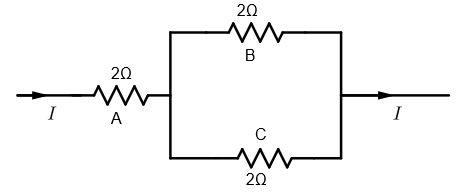Here, P = 18 W
Since A is in  series with the parallel combination of B and C. So, it carries maximum current.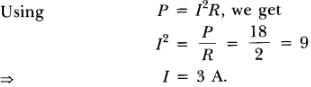Let IB and IC be the current flowing through B and C respectively. As they are in parallel, potential difference across them will be same, so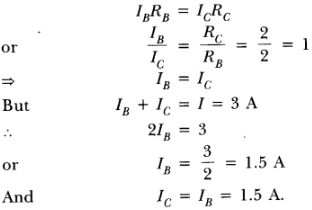Ques 2: Should the resistance of an ammeter be low or high? Give reason.
Ans: The resistance of an ammeter should be low so that it will not disturb the magnitude of current flowing through the circuit when connected in series in a circuit.

Ques 3: How does use of a fuse wire protect electrical appliances?
Ans: The fuse wire is always connected in series with the live wire or electrical devices. If the flow of current exceeds the specified preset value due to some reason, the heat produced melts it and disconnects the circuit or the device from the mains. In this way, fuse wire protects the electrical appliances.

Ques 4: What is electrical resistivity? In a series electrical circuit comprising a resistor made up of a metallic wire, the ammeter reads 5 A. The reading of the ammeter decreases to half when the length of the wire is doubled. Why?
Ans: The resistance offered by a metallic wire of unit length and unit cross-sectional area is called electrical resistivity.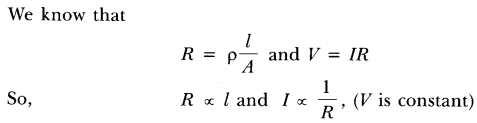Hence, when the length of wire is doubled, the resistance becomes double and current decreases to half.

Ques 5: A current of 1 ampere flows in a series circuit containing an electric lamp and a conductor of 5 Ω when connected to a 10 V battery. Calculate the resistance of the electric lamp.
Now if a resistance of 10 Ω is connected in parallel with this series combination, what change (if any) in current flowing through 5 Ω conductor and potential difference across the lamp will take place? Give reason.
Ans:
Given: In series circuit containing  lamp and resistor,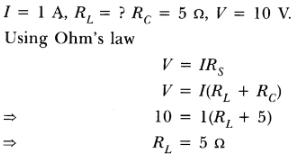So, resistance of an electrical lamp is 5Ω.
According to the given condition, circuit can be redrawn as shown.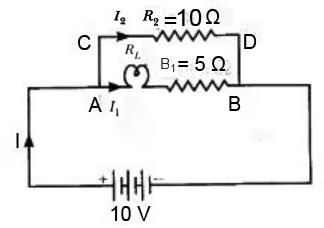So, effective resistance of parallel combination.Current drawn form the battery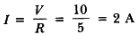As in parallel combination potential difference across them remains same, So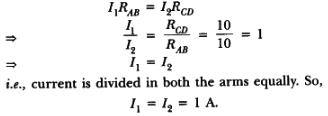Hence, there will be no change in the current through 5Ω conductor. Also there will be no change in the potential difference across the lamp as in both cases, current through the lamp remains same i.e. 1A.

Ques 6: Why is parallel arrangement used in domestic wiring?
Ans: Parallel arrangement is used in domestic wiring because
(i) Each appliance gets the same voltage as that of the mains supply.
(ii) If one component is switched off, others can work properly.
(iii) Fault in any branch of the circuit can be easily identified.

Que 7: B1, B2 and B3 are three identical bulbs connected as shown in figure. When all the three bulbs glow, a current of 3A is recorded by the ammeter A.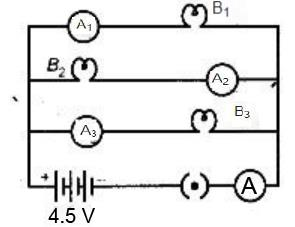(i) What happens to the glow of the other two bulbs when the bulb B j gets fused?
(ii) What happens to the reading of A1 ,A2 , A3 and A when the bulb B2 gets fused?
(iii) How much power is dissipated in the circuit when all the three bulbs glow together?

Ans: (i) Since B1 ,B2 and B3 are in parallel, the potential difference across each of them will remain same. So when the bulb B1 gets fused, B2 and B3 have the same potential and continues with the same energy dissipated per second, i.e. they will glow continuously as they were glowing before.
(ii) Resistance of the parallel combination when all the three bulbs are glowing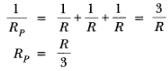Ammeter 'A' reads 3 A current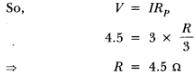So, resistance of each bulb =  4.5 Ω.
Now when bulb Bgets fused, the equivalent resistance of parallel combination of B1 and Bis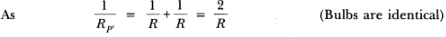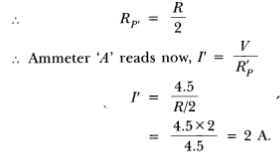Since resistance of each arm is same and p.d. is also same, current divides them equally. So 1 A current will pass through each bulb B1 and B3.
Therefore, ammeter A1 and A3  reads 1 current while A2 will read zero and ammeter A read 2 A current
(iii) In parallel. total power consumed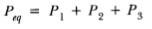So, when all the three bulbs glow together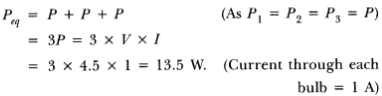Ques 8: Three incandescent bulbs of 100 W each are connected in series in an electric circuit. In another circuit, another set of three bulbs of the same wattage are connected in parallel to the same source.
(а) Will the bulb in the two circuits glow with the same brightness? Justify your answer.
(b) Now let one bulb in both the circuits get fused. Will the rest of the bulbs continue to glow in each circuit? Give reason.

Ans: For three identical bulbs,
In series, RS = 3R
In Parallel, Rp = R/3.
(a) The bulbs in the two circuits will not glow equally bright as the current through them is not the same.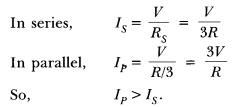(b) As one bulb fuses, the other bulbs in the series circuit will not glow because the circuit becomes an open circuit. While the rest of bulbs in parallel circuit will continue to glow without getting disturbed because in parallel combination, current gets additional paths to flow.

Ques 9: Find out the following in the electric circuit given in figure: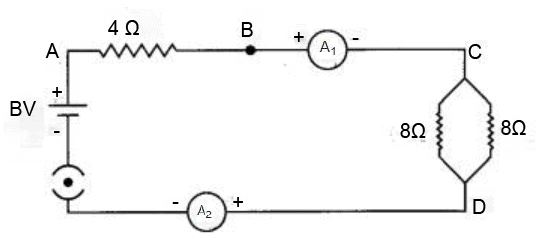Ans:
(a) Effective resistance, the two 8Ω resistors in parallel,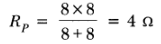(b) Req = 4 + Rp = 8Ω
So current through 4Ω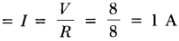(c) Potential difference across resistance 4Ω = V1 = IR = 1 * 4 = 4 V
(d) Power dissipated = I2R = I2 * 4 = 4 W
(e) No difference, since the ammeters are connected in series and same current will pass through them, so reading of both ammeters will be same.

Offer running on EduRev: Apply code STAYHOME200 to get INR 200 off on our premium plan EduRev Infinity!

## Science Class 10

84 videos|257 docs|129 tests

,

,

,

,

,

,

,

,

,

,

,

,

,

,

,

,

,

,

,

,

,

;